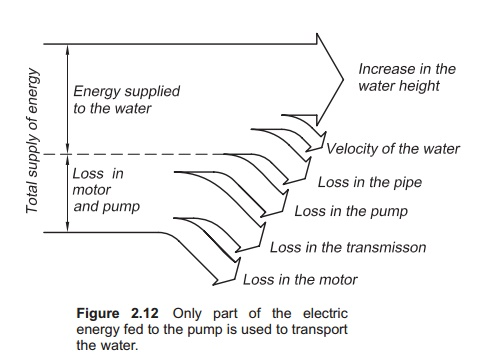Home | | Aquaculture Engineering | Pumping of water requires energy

# Pumping of water requires energy

Energy is required to pump water from one level to another. Energy consumption is usually expressed as power (P), which is energy supplied per unit time. P is measured in joules per second; 1 J/s = 1 watt (W).

Pumping of water requires energy

Energy is required to pump water from one level to another. Energy consumption is usually expressed as power (P), which is energy supplied per unit time. P is measured in joules per second; 1 J/s = 1 watt (W).

The following equation can be used to calculate the energy requirement for pumping:

= ρghQ

where:

ρ = density of water (kg/m3)

=acceleration due to gravity (m/s2) Q =water flow rate (m3/s)

h height that the water is pumped.

Example

Calculate the energy required to lift 1000 l/min of water by 5 m and 15 m (including the friction head). The density of water is 1025 kg/m3, the flow rate is 0.016 m3/s and acceleration due to gravity is 9.81 m/s2.

Case 1: 5 m lift

= ρ ghQ

= 1025 kg/m3× 9.81 m/s2× 5 m × 0.016 m3/s

=

=804.4 J/s

=804.4 W.

Case 2: 15 m lift

= ρghQ

=1025 kg/m3× 9.81 m/s2× 15 m × 0.016 m3/s

=2413.3 W

=2.4 kW.

This illustrates that by tripling the pump height the energy requirement is also tripled.

The power supplied from the pump to the water is called the water effect and is the sum of the veloc-ity head, head loss and static head. As a result of energy losses in the pump, more power is supplied to the pump than the pump supplies to the water. The efficiency of the pump, is given by the equation

η = PD/PS

where:

η efficiency

PD=energy delivered from the pump to the water

PS=energy supplied to the pump.

Example

A pump has an efficiency of 75% and consumes 5 kW of electric power. How much of this power is used to pump the water?

η = PD/PSPD=hPS

=0.75 × 5

=3.75 kWEnergy losses occur at several places in the pump. This results in efficiencies below unity (Fig. 2.12). Losses occur in the pump motor (m), trans-mission (t) and in the impeller (p), the sum of which gives the pump efficiency. Low pump efficiency results in the creation of heat, because energy cannot disappear; for example, when using sub-merged pumps this energy will be transferred to the water, which is heated. In water re-use systems this heating can be noticeable. The total efficiency of a pump η A, can be calculated as follows:

η A  = η p  + η t  + η m

Efficiency may also be defined as hydraulic efficiency ηH (loss when the water flows through a pump), volumetric efficiency ηv (leakage of water between suction and pressure side of the pump, for example in centrifugal pumps) and mechanical efficiency η m (losses in the motor and transmission).

In fish farming the usual efficiency of well-suited pumps is around 0.7. Efficiency normally varies between 0.4 and 0.8.

## Pump costs

To calculate the cost of pumping, the following equation can be used:

Pumping cost =PdEP

where:

P power (kW)

duration of pumping (h)

EP = electricity price per kWh (kilowatt per hour).

Example

A centrifugal pump with a 5 kW power supply runs continuously to supply a fish farm with water. Cal-culate the yearly electricity cost of running the pump with an electricity price of 0.1 €.

Yearly pumping cost =PdEP

= 5 kW × 24 h × 365 days ×  €/kWh

=4380 €.

Study Material, Lecturing Notes, Assignment, Reference, Wiki description explanation, brief detail
Aquaculture Engineering : Water Transport : Pumping of water requires energy |